# dissect

## 语法

``p = dissect(A)``
``p = dissect(A,Name,Value)``

## 说明

``p = dissect(A)` 返回一个使用 `A` 的稀疏结构的嵌套剖分计算的置换向量。`

``p = dissect(A,Name,Value)` 使用一个或多个名称-值对组参数指定其他选项。例如，`dissect(A,'NumIterations',15)` 在嵌套剖分算法中使用 15 次细化迭代，而不是 10 次。`

## 示例

```load west0479.mat A = west0479; spy(A)```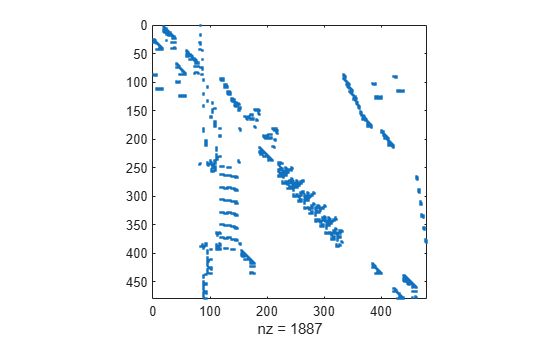```p1 = dissect(A); p2 = amd(A); p3 = symrcm(A);```

```subplot(1,2,1) spy(A) title('Original Matrix') subplot(1,2,2) spy(lu(A)) title('LU Decomposition')```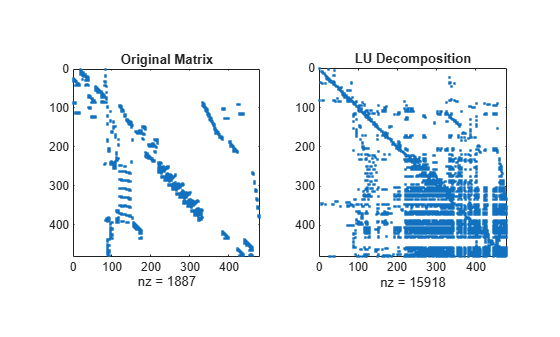```figure subplot(1,2,1) spy(A(p3,p3)) title('Reverse Cuthill-McKee') subplot(1,2,2) spy(lu(A(p3,p3))) title('LU Decomposition')```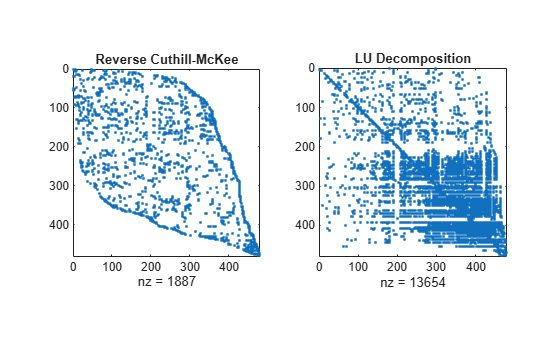```figure subplot(1,2,1) spy(A(p2,p2)) title('Approximate Minimum Degree') subplot(1,2,2) spy(lu(A(p2,p2))) title('LU Decomposition')```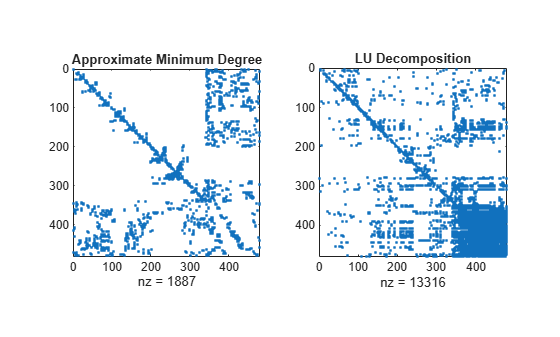```figure subplot(1,2,1) spy(A(p1,p1)) title('Nested Dissection') subplot(1,2,2) spy(lu(A(p1,p1))) title('LU Decomposition')``````A = speye(100) + diag(ones(1,99),1) + diag(ones(1,98),2); A(1:5,:) = ones(5,100); A = A + A'; spy(A)```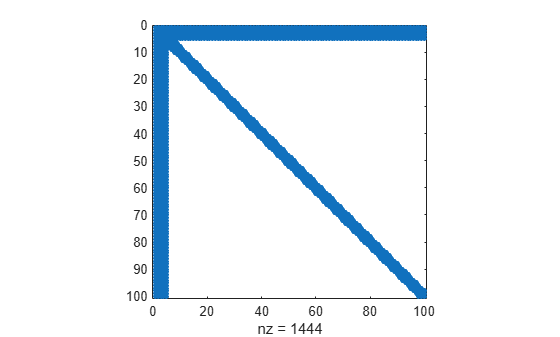`p = dissect(A,'MaxDegreeThreshold',10);`

`spy(A(p,p))`## 算法

 中介绍的嵌套剖分排序算法是一种多级图形分区算法，用于对稀疏矩阵进行减少填充量的排序。输入矩阵被视为图形的邻接矩阵。该算法通过折叠顶点和边来粗化图形，重排较小的图形，然后通过细化步骤对较小图形去粗，得到重排的原始图形。

`dissect` 的名称-值对组使您能够控制算法的各个阶段：

• 粗化

在此阶段，算法将相邻的顶点对组折叠，从原始图生成连续的更小的图。您可以借助 `'MaxDegreeThreshold'`，最后再对高度连接的图顶点（矩阵中的密集列）进行排序，以将它们过滤出来。

• 分区

在图形粗化后，算法对更小的图形彻底重新排序。在每个分区步骤中，算法尝试将图形分成相等的部分：`'NumSeparators'` 指定将图形分成多少部分，`'VertexWeights'` 为顶点分配权重（可选），而 `'MaxImbalance'` 指定不同分区之间权重差的阈值。

• 细化

在对最小的图形重新排序后，算法展开之前合并的顶点进行投影，将图形放大到原始大小。在每个投影步骤后，执行细化步骤，移除分区之间的顶点，以提高解的质量。`'NumIterations'` 控制该去粗阶段使用的细化步骤的数量。

 Karypis, George and Vipin Kumar. "A Fast and High Quality Multilevel Scheme for Partitioning Irregular Graphs." SIAM Journal on Scientific Computing. Vol. 20, Number 1, 1999, pp. 359–392.